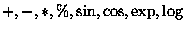Next: Results Up: Grammatical Evolution : Evolving Previous: Pruning

# The Problem Space

As proof of concept, we have applied our system to a symbolic regression problem used by Koza [Koza 92]. The system is given a set of input and output pairs, and must determine the function that maps one onto the other. The particular function Koza examined is X4 + X3 + X2 + Xwith the input values in the range [-1..+1]. Table  4 contains a tableau which summarizes his experiments.

Table 4: A Koza-style tableau
 Objective : Find a function of one independant variable and one dependant variable, in symbolic form that fits a given sample of 20 (xi, yi) data points, where the target functions is the quartic polynomial X4 + X3 + X2 + X GPTerminal Set: X (the independant variable) GPFunction SetFitness cases The given sample of 20 data points in the interval [-1, +1] Raw Fitness The sum, taken over the 20 fitness cases, of the error Standardised Fitness Same as raw fitness Hits The number of fitness cases for which the error is less than 0.01 Wrapper None Parameters M = 500, G = 51

The production rules for <expr> are given above. As this and subsequent rules are the only ones that require a choice, they are the ones that will be evolved.

We adopt a similar style to Koza of summarizing information, using a modified version of his tableau. Notice how our terminal operands and terminal operators are analgous to GPTerminals and GPfunctions respectively.

Table 5: Grammatical Evolution Tableau
 Objective : Find a function of one independant variable and one dependant variable, in symbolic form that fits a given sample of 20 (xi, yi) data points, where the target functions is the quartic polynomial X4 + X3 + X2 + X Terminal Operands: X (the independant variable) Terminal Operators The binary operators +, *, /, and - The unary operators Sin, Cos, Exp and Log Fitness cases The given sample of 20 data points in the interval [-1, +1] Raw Fitness The sum, taken over the 20 fitness cases, of the error Standardised Fitness Same as raw fitness Hits The number of fitness cases for which the error is less than 0.01 Wrapper Standard productions to generate C functions Parameters M = 500, G = 51Next: Results Up: Grammatical Evolution : Evolving Previous: Pruning
Red Hat Linux User
1998-10-02